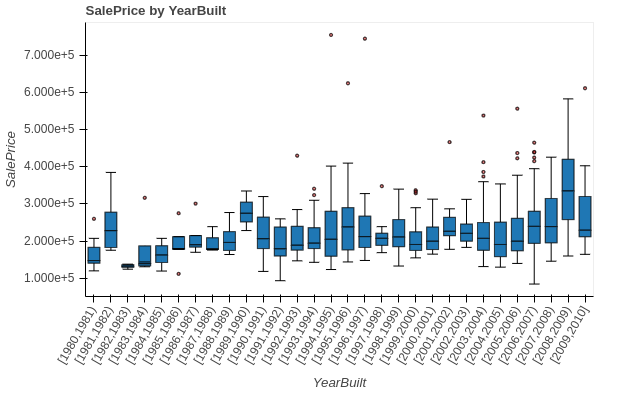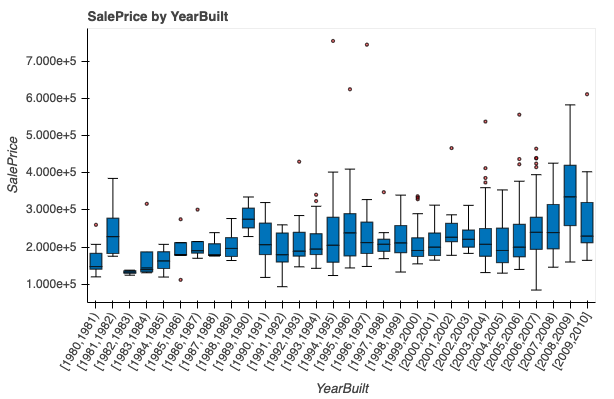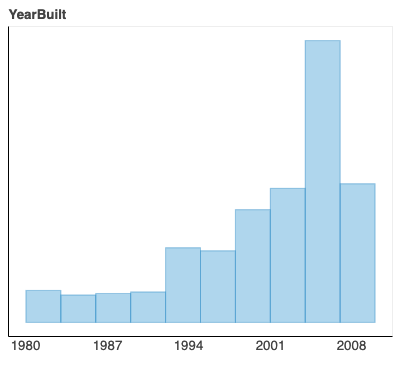# Force the xaxis to be linear within an interval

I currently have this plotI don’t want there to be one tick per boxplot on the xaxis. Rather, I want to make the xaxis linear where I specify the lower and upper bounds on the range of the xaxis values, and then I want to specify a few ticks in that range that don’t necessarily line up with the center of a box plot. Is this possible?

The code to build this visualization is similar to this example https://docs.bokeh.org/en/latest/docs/gallery/boxplot.html.

Thanks

It sounds like you should specify all the ticks explicitly yourself. You can do that with an explicit `FixedTicker`, or as a convenience, by passing a list of ticks directly:

``````p.yaxis.ticker = [<list of tick locations>]
``````

Thanks for the reply. This is a possible solution, but I would like to override the xaxis tick values with dates. I added the following two lines to the end of the boxplot example from my previous post, but the override isn’t working:

``````p.xaxis.ticker = [1,2] # this works
p.xaxis.major_label_overrides = {1:"1980"} # this override does nothing
``````

Thanks for any help with this

Without the code, it’s impossible to tell what’s wrong. `major_label_overrides` works just fine for me.

Sorry. The code is

``````import numpy as np
import pandas as pd

from bokeh.plotting import figure, output_file, show

# generate some synthetic time series for six different categories
cats = list("abcdef")
yy = np.random.randn(2000)
g = np.random.choice(cats, 2000)
for i, l in enumerate(cats):
yy[g == l] += i // 2
df = pd.DataFrame(dict(score=yy, group=g))

# find the quartiles and IQR for each category
groups = df.groupby('group')
q1 = groups.quantile(q=0.25)
q2 = groups.quantile(q=0.5)
q3 = groups.quantile(q=0.75)
iqr = q3 - q1
upper = q3 + 1.5*iqr
lower = q1 - 1.5*iqr

# find the outliers for each category
def outliers(group):
cat = group.name
return group[(group.score > upper.loc[cat]['score']) | (group.score < lower.loc[cat]['score'])]['score']
out = groups.apply(outliers).dropna()

# prepare outlier data for plotting, we need coordinates for every outlier.
if not out.empty:
outx = []
outy = []
for keys in out.index:
outx.append(keys)
outy.append(out.loc[keys].loc[keys])

p = figure(tools="", background_fill_color="#efefef", x_range=cats, toolbar_location=None)

# if no outliers, shrink lengths of stems to be no longer than the minimums or maximums
qmin = groups.quantile(q=0.00)
qmax = groups.quantile(q=1.00)
upper.score = [min([x,y]) for (x,y) in zip(list(qmax.loc[:,'score']),upper.score)]
lower.score = [max([x,y]) for (x,y) in zip(list(qmin.loc[:,'score']),lower.score)]

# stems
p.segment(cats, upper.score, cats, q3.score, line_color="black")
p.segment(cats, lower.score, cats, q1.score, line_color="black")

# boxes
p.vbar(cats, 0.7, q2.score, q3.score, fill_color="#E08E79", line_color="black")
p.vbar(cats, 0.7, q1.score, q2.score, fill_color="#3B8686", line_color="black")

# whiskers (almost-0 height rects simpler than segments)
p.rect(cats, lower.score, 0.2, 0.01, line_color="black")
p.rect(cats, upper.score, 0.2, 0.01, line_color="black")

# outliers
if not out.empty:
p.circle(outx, outy, size=6, color="#F38630", fill_alpha=0.6)

p.xgrid.grid_line_color = None
p.ygrid.grid_line_color = "white"
p.grid.grid_line_width = 2
p.xaxis.major_label_text_font_size="12pt"

# changes here
p.xaxis.ticker = [1,2]
p.xaxis.major_label_overrides = {1:"1980"}

show(p)
``````

The output is

The expected output is for the xaxis tick value 1 to be overridden by “1980”.

I’m using bokeh version 2.0.0. Thanks

So, I would say that `p.xaxis.ticker = [1,2] ` doing anything in particular with a categorical axis is accidental. There is a synthetic numeric coordinate system that underlies categorical axes, but this is not really exposed to users. I guess I am not surprised that this “works” but it is definitely not intentional that it behaves this way. I am not actually sure that this situation with tick overrides for categorical axes has ever been explicitly considered. What happens if you use actual (string) categorical coordinates for the ticker and tick overrides?

Using` p.xaxis.major_label_overrides` on the categorical coordinates doesn’t work. The following code

``````import numpy as np
import pandas as pd

from bokeh.plotting import figure, output_file, show

# generate some synthetic time series for six different categories
cats = list("abcdef")
yy = np.random.randn(2000)
g = np.random.choice(cats, 2000)
for i, l in enumerate(cats):
yy[g == l] += i // 2
df = pd.DataFrame(dict(score=yy, group=g))

# find the quartiles and IQR for each category
groups = df.groupby('group')
q1 = groups.quantile(q=0.25)
q2 = groups.quantile(q=0.5)
q3 = groups.quantile(q=0.75)
iqr = q3 - q1
upper = q3 + 1.5*iqr
lower = q1 - 1.5*iqr

# find the outliers for each category
def outliers(group):
cat = group.name
return group[(group.score > upper.loc[cat]['score']) | (group.score < lower.loc[cat]['score'])]['score']
out = groups.apply(outliers).dropna()

# prepare outlier data for plotting, we need coordinates for every outlier.
if not out.empty:
outx = []
outy = []
for keys in out.index:
outx.append(keys)
outy.append(out.loc[keys].loc[keys])

p = figure(tools="", background_fill_color="#efefef", x_range=cats, toolbar_location=None)

# if no outliers, shrink lengths of stems to be no longer than the minimums or maximums
qmin = groups.quantile(q=0.00)
qmax = groups.quantile(q=1.00)
upper.score = [min([x,y]) for (x,y) in zip(list(qmax.loc[:,'score']),upper.score)]
lower.score = [max([x,y]) for (x,y) in zip(list(qmin.loc[:,'score']),lower.score)]

# stems
p.segment(cats, upper.score, cats, q3.score, line_color="black")
p.segment(cats, lower.score, cats, q1.score, line_color="black")

# boxes
p.vbar(cats, 0.7, q2.score, q3.score, fill_color="#E08E79", line_color="black")
p.vbar(cats, 0.7, q1.score, q2.score, fill_color="#3B8686", line_color="black")

# whiskers (almost-0 height rects simpler than segments)
p.rect(cats, lower.score, 0.2, 0.01, line_color="black")
p.rect(cats, upper.score, 0.2, 0.01, line_color="black")

# outliers
if not out.empty:
p.circle(outx, outy, size=6, color="#F38630", fill_alpha=0.6)

p.xgrid.grid_line_color = None
p.ygrid.grid_line_color = "white"
p.grid.grid_line_width = 2
p.xaxis.major_label_text_font_size="12pt"

p.xaxis.major_label_overrides = {"a":"1980"}

show(p)
``````

Gives this result

Just to clarify, for a visualization like thisI want to override the xaxis, tick values, and their locations to have an xaxis and tick values like in this plotI’m sure this is an obscure request, I’m just curious if it’s possible. Thanks

@blockhart I understand what you want, but it should be pointed out that the two plots you show are fundamentally different. The first has a categorical axis, and the second is a histogram with a continuous numerical axis. All of the tick override machinery has so far only be developed/tested with the latter.

So there are a few other options.

• You could could keep all the normal ticks, but use `FuncTickFomatter` to display an empty string for some of the tick labels. Practically speaking, that seems like it would have the visual effect you want.

• You could create a custom extension ticker that filters out ticks you don’t want. That’s a bit involved, but here is a sample that discards every other tick:

``````JS_CODE = """
import {CategoricalTicker, FactorTickSpec} from "models/tickers/categorical_ticker"
import {FactorRange} from "models/ranges/factor_range"

export class MyTicker extends CategoricalTicker {

get_ticks(start: number, end: number, range: FactorRange, cross_loc: any, _: any): FactorTickSpec {
const ticks = super.get_ticks(start, end, range, cross_loc, _)

function filt(_: any, index: number, __: any) {
return (index % 2 == 0)
}
ticks.major = ticks.major.filter(filt)

return ticks
}
}
"""

class MyTicker(CategoricalTicker):
__implementation__ = JS_CODE

fruits = ['Apples', 'Pears', 'Nectarines', 'Plums', 'Grapes', 'Strawberries']

p = figure(x_range=fruits, plot_height=250, title="Fruit Counts",
tools="xwheel_zoom")

p.xaxis.ticker = MyTicker()
``````

Thanks a lot for your help with this, `FuncTickFormatter` gave me the effect I wanted.

1 Like